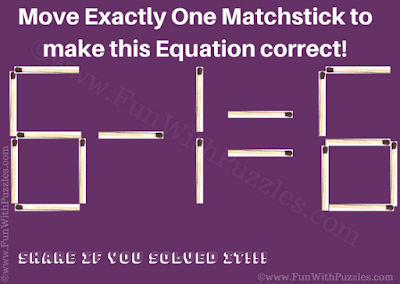This is very interesting and easy Matchstick Picture Puzzle for kids. Thanks to "Ansh Gupta" for helping me to create this Matchstick Picture puzzle. In this Puzzle Picture, there is Mathematically equation created with matchsticks. This equation is not correct. Your challenge is to move only one matchstick and make this equation correct. Let's see if you can solve this easy matchstick puzzle?Move exactly one matchstick to make equation correct?

The answer to this "Matchstick Puzzle for Kids", can be viewed by clicking on the button. Please do give your best try before looking at the answer.